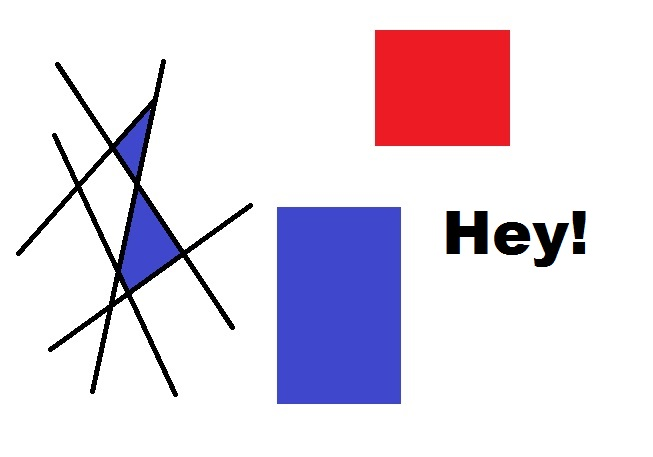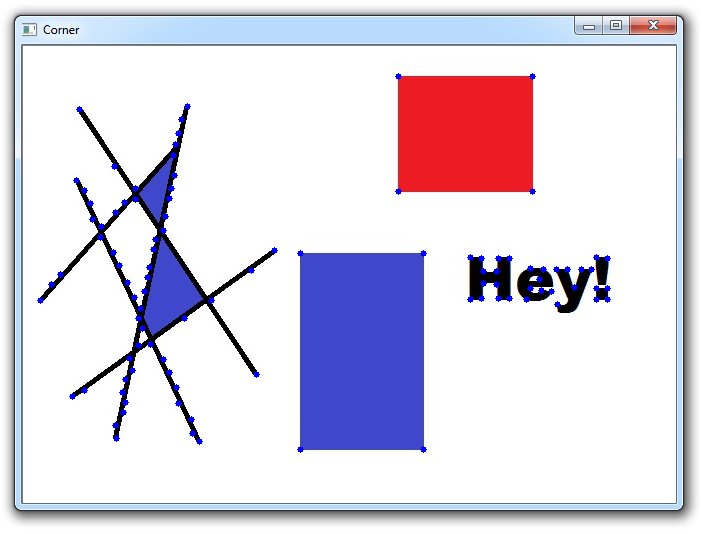## Corner Detection OpenCV Python Tutorial

Welcome to a corner detection with OpenCV and Python tutorial. The purpose of detecting corners is to track things like motion, do 3D modeling, and recognize objects, shapes, and characters.

For this tutorial, we're going to use the following image:Our goal here is to find all of the corners in this image. I will note that we have some aliasing issues here (jagged-ness in slanted lines), so, if we let it, a lot of corners will be found, and rightly-so. As usual with OpenCV, the hard part is done for us already, and all we need to do is feed in some parameters. Let's start with loading the image and setting some parameters:

```import numpy as np
import cv2

gray = cv2.cvtColor(img,cv2.COLOR_BGR2GRAY)
gray = np.float32(gray)

corners = cv2.goodFeaturesToTrack(gray, 100, 0.01, 10)
corners = np.int0(corners)```

So far, we load the image, convert to gray, then to float32. Next, we detect corners with the goodFeaturesToTrack function. The parameters here are the image, max corners to detect, quality, and minimum distance between corners. As mentioned before, the aliasing issues we have here will allow for many corners to be found, so we put a limit on it. Next:

```for corner in corners:
x,y = corner.ravel()
cv2.circle(img,(x,y),3,255,-1)

cv2.imshow('Corner',img)```

Now we iterate through each corner, making a circle at each point that we think is a corner.In the next tutorial, we're going to be discussing feature matching/homography.

The next tutorial:• Drawing and Writing on Image OpenCV Python Tutorial

• Image Operations OpenCV Python Tutorial

• Image arithmetics and Logic OpenCV Python Tutorial

• Thresholding OpenCV Python Tutorial

• Color Filtering OpenCV Python Tutorial

• Blurring and Smoothing OpenCV Python Tutorial

• Morphological Transformations OpenCV Python Tutorial

• Canny Edge Detection and Gradients OpenCV Python Tutorial

• Template Matching OpenCV Python Tutorial

• GrabCut Foreground Extraction OpenCV Python Tutorial

• Corner Detection OpenCV Python Tutorial
• Feature Matching (Homography) Brute Force OpenCV Python Tutorial

• MOG Background Reduction OpenCV Python Tutorial

• Haar Cascade Object Detection Face & Eye OpenCV Python Tutorial